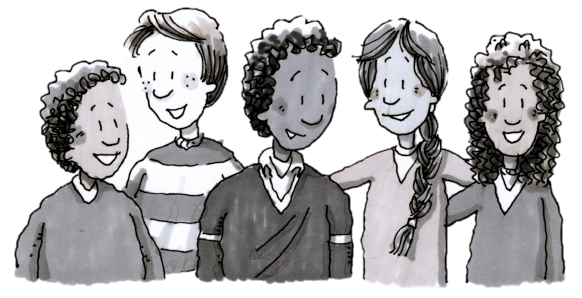### Home > MC1 > Chapter 1 > Lesson 1.3.3 > Problem1-122

1-122.

There are five students of different ages whose median age is $13$ years.

1. What are two possibilities for the ages of the three oldest students?

If we know that $13$ is the median age, what do we know about the set of numbers? Do you know where $13$ would fall in the set of five numbers?

2. If each student is a different age, how many students must be younger than $13$?

Remember that to find the median, it is helpful to put numbers in order from least to greatest.

Two students must be younger than $13$. Because $13$ is the median age, we know that the sequence looks something like this: ?, ?, $13$, ?, ?. Because the numbers are arranged from least to greatest, we know that the two students represented to the left of $13$ are younger.

3. In complete sentences, describe what a median is to another student in the class. Pretend that the student was absent the day you learned about medians.

It may be helpful to look at the definition of median in your textbook on page $54$.